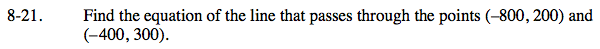### Home > CAAC > Chapter 8 > Lesson 8.1.2 > Problem8-21

8-21.

Find the equation of the line that passes through the points (−800, 200) and (−400, 300). 8-21 HW eTool (Desmos). Homework Help ✎Find the growth.

$\text{Slope (growth) }=\frac{\text{change in }y}{\text{change in }x}=\frac{100}{400}$

$\textit{m}=\frac{1}{4}$

Substitute the growth (m) and a point (x, y) from the problem into y = mx + b. Solve for b.

$200=\frac{1}{4}(-800)+\textit{b}$

200 = −200 + b

400 = b

$\textit{y}=\frac{1}{4}\textit{x}+400$